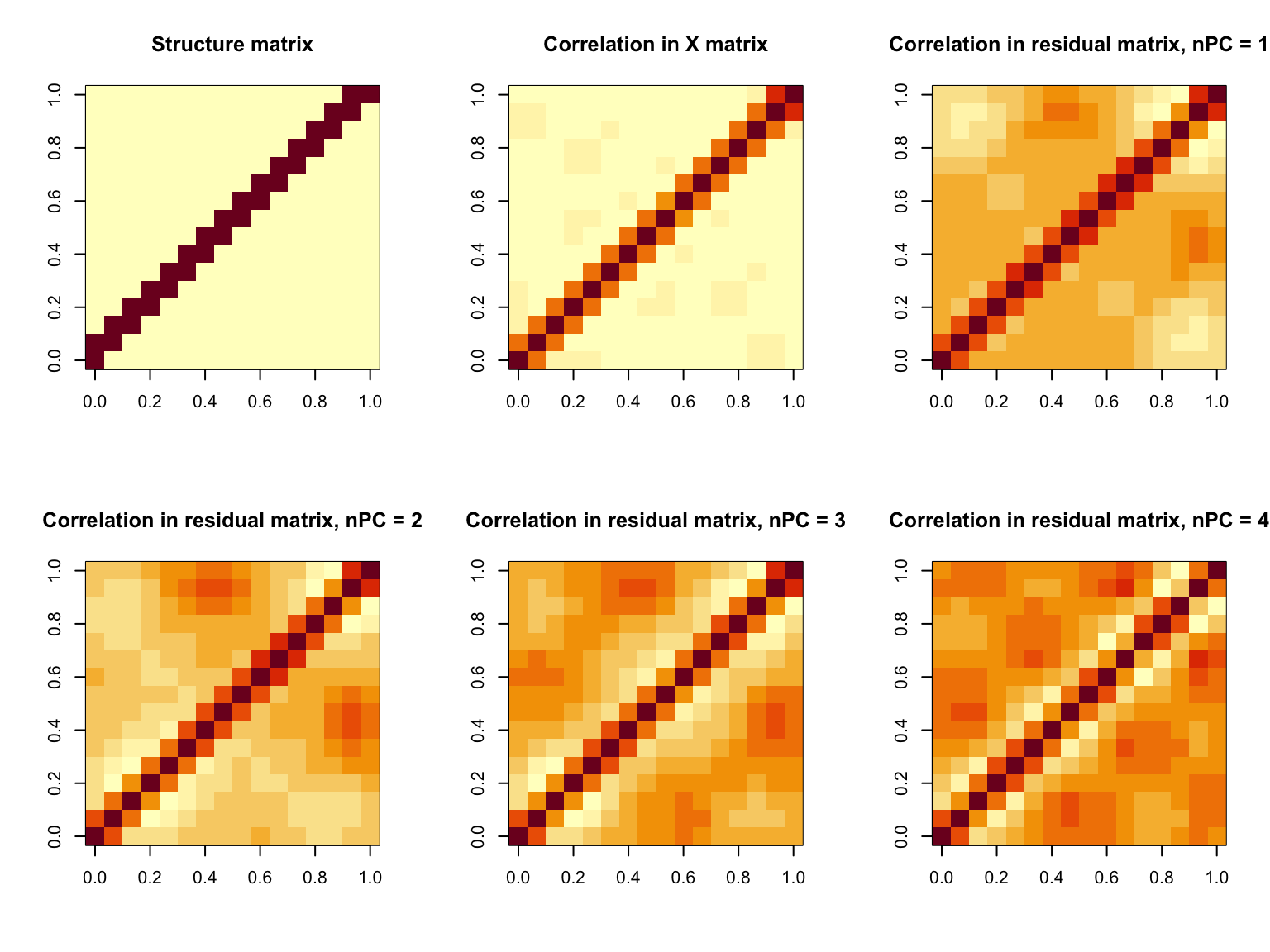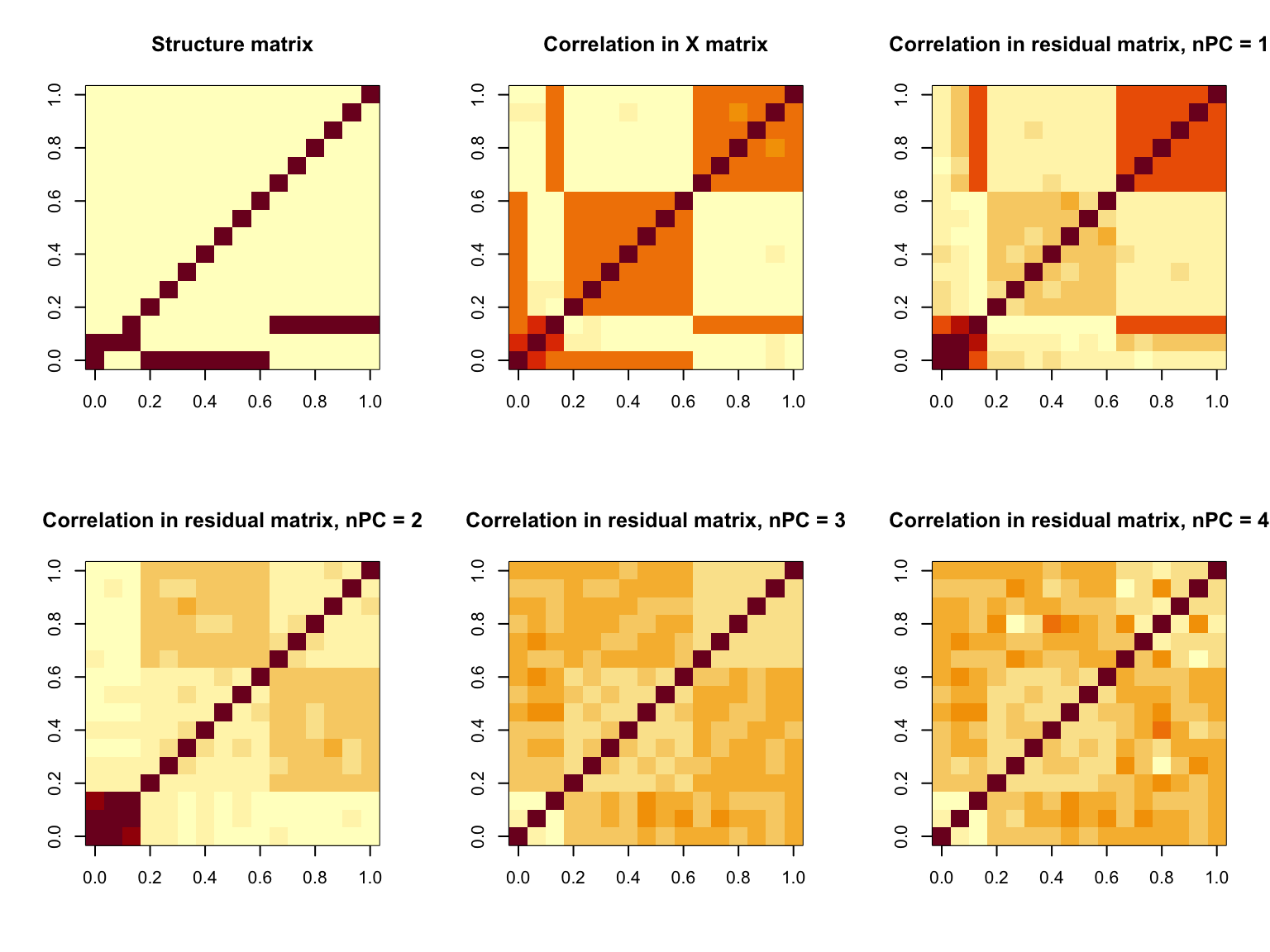# Exploration on regressing out PCs

Some going-to-nowhere exploration on regressing out PCA
Author

Yanyu Liang

Published

November 4, 2020

$\newcommand{\var}{\text{Var}} \newcommand{\E}{\text{E}} \newcommand{\diag}{\text{diag}} \newcommand{\cov}{\text{Cov}}$

Recently, we encountered a data matrix with correlated columns. To account for this, we thought about performing SVD on the matrix and then regressing out the left singular vectors from the columns (or equivalently regressing out the right singular vectors from the rows).

It turns out that we could removing a lot of correlation after regressing out top N PCs (which captures about 50% PVE). But the issue is that this approach is not removing all of the correlations. There are some left over correlations that cannot be removed by PCA (in our case we ended up having block wise diagonal correlation).

And if we focus on the block of features that have remaining correlation and perform PCA for them, we cannot furthre remove any correlation anymore.

Now that the question is why PCA works at first but fails afterwards. And more specifically, we want to know with what data pattern/characteristic, the PCA approach works and when it could not work out.

Here, I do a very simple exploration on this problem. The arguments to deliver are the following:

• When regressing out PCs removes the correlation structure, it captures the common factors shared by almost all features.
• When the features do not share common factor, this approach could fail.

Here we do a super simple simulation. Suppose we have $$K = 16$$ hidden factors $$z_1, \cdots, z_K$$. The factor sharing pattern is governed by a structure matrix $$S$$. For each feature $$x_{ij} = \sum_k S_{kj} z_{ik}$$ and the data matrix is formed by $$X = Z S$$ and we let $$z_{ik} \sim N(0, 1)$$. Here we impose two types of $$S$$ and, in both cases, try to regress out the first PC from the data matrix $$X = Z S$$.

Show the code
set.seed(2020)
standardize = function(x) {
apply(x, 2, function(y) { (y - mean(y)) / sd(y) })
}
split_by_pca = function(x, pve_cutoff = 0.5, npc = NULL) {
x = standardize(x)
res = svd(x)
v = res$v if(is.null(npc)) { pve = cumsum(res$d^2 / sum(res$d^2)) npc = sum(pve <= pve_cutoff) + 1 } pc_mat = res$u[, 1 : npc, drop = F]
res = x - pc_mat %*% (t(pc_mat) %*% x)
list(residual = res, pc = pc_mat, v = v)
}
k = 16
n = 1000
struct_mat = matrix(0, nrow = k, ncol = k)
for(j in 1 : k) {
struct_mat[j, j] = 1
if(j < k) {
struct_mat[j, j + 1] = 1
}
if(j > 1) {
struct_mat[j - 1, j] = 1
}
}
struct_mat2 = matrix(0, nrow = k, ncol = k)
struct_mat2[4 : 10, 1] = 1
struct_mat2[1:3, 2] = 1
struct_mat2[11:k, 3] = 1
for(j in 1 : k) {
struct_mat2[j, j] = 1
}
zz = matrix(rnorm(n * k), nrow = n)
x = zz %*% t(struct_mat)
x2 = zz %*% t(struct_mat2)
tmp = split_by_pca(x, npc = 1)
res = tmp$residual par(mfrow=c(2,3)) image(struct_mat, main = 'Structure matrix') image(cor(x), main = 'Correlation in X matrix') for(k in 1 : 4) { tmp = split_by_pca(x, npc = k) res = tmp$residual
image(cor(res), main = paste0('Correlation in residual matrix, nPC = ', k))
}Show the code
par(mfrow=c(2,3))
image(struct_mat2, main = 'Structure matrix')
image(cor(x2), main = 'Correlation in X matrix')
for(k in 1 : 4) {
tmp2 = split_by_pca(x2, npc = k)
res2 = tmp2\$residual
image(cor(res2), main = paste0('Correlation in residual matrix, nPC = ', k))
}